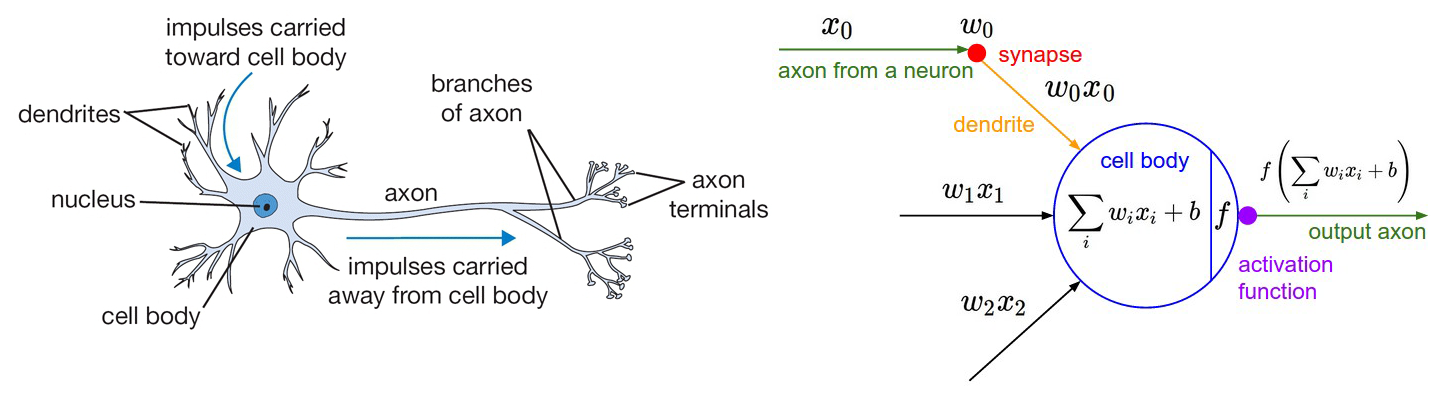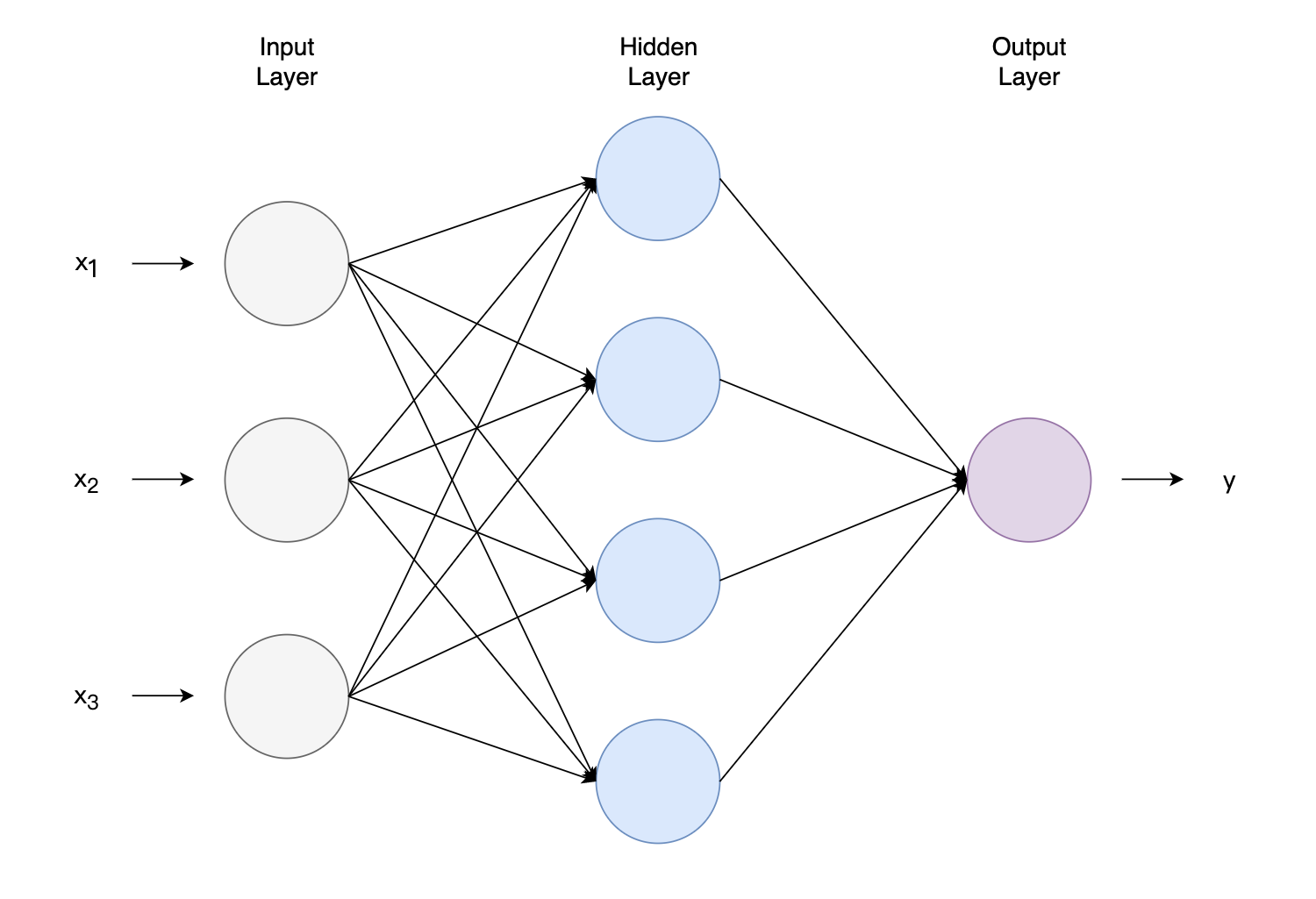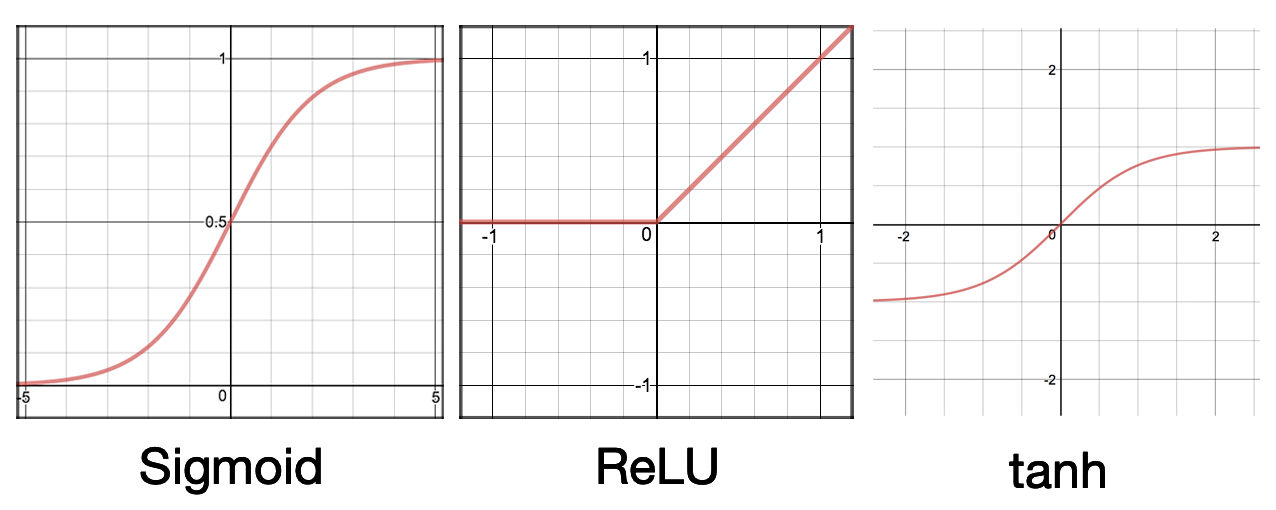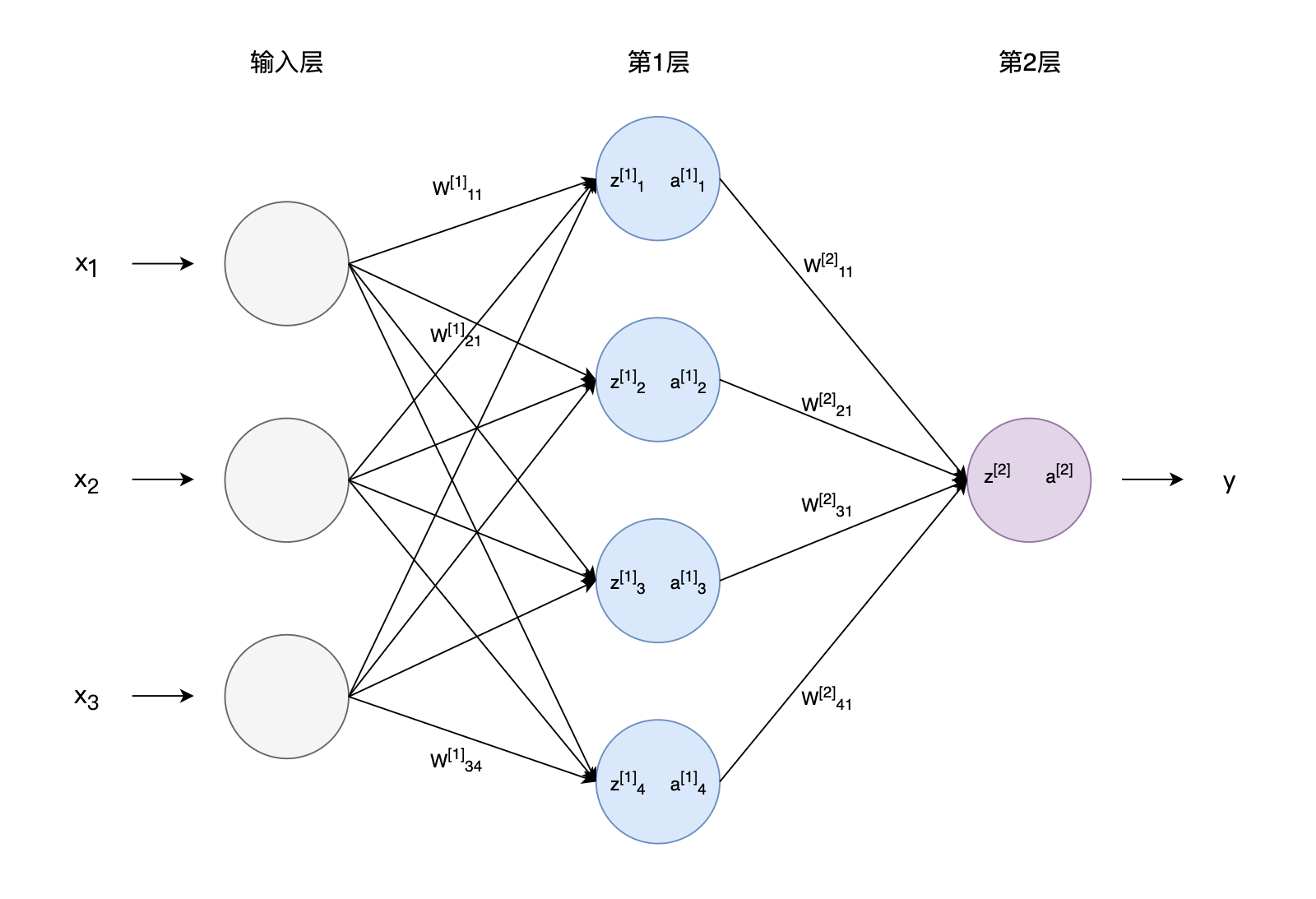# 前馈神经网络

## 神经元模型与神经网络图1 神经元模型图2 神经网络图3 常见激活函数

Sigmoid函数或者又称为Logistic函数，我们曾在Logistic Regression中介绍过，它将$(-\infty, +\infty)$的输入压缩到了0和1之间。经过Sigmoid函数后，较大的负数会接近0，较大的正数会接近1。Sigmoid函数的这些特征可以很好地解释神经元模型，0表示不产生兴奋，1表示产生兴奋。早期的神经网络喜欢使用Sigmoid函数作为激活函数，但Sigmoid函数也有一些弊端，现在的神经网络几乎不再使用它了。Logistic Regression使用了Sigmoid函数，Logistic Regression又被认为是一种非常简单的神经网络。

## 前馈神经网络

### 向量化表示

$z_{1}^{} = {W_1^{}}^T\boldsymbol{x} +b_1^{} \quad \quad a_{1}^{} = g(z_{1}^{}) \\ \vdots \\ z_{4}^{} = {W_4^{}}^T\boldsymbol{x} +b_4^{} \quad \quad a_{4}^{} = g(z_{4}^{})$

$\boldsymbol{a}^{} = \begin{bmatrix} a_{1}^{} \\ a_{2}^{} \\ a_{3}^{} \\ a_{4}^{} \end{bmatrix}$ 将这个向量$\boldsymbol{a}^{}$发送到输出层：

$z^{} = {W^{}}\boldsymbol{a}^{} +b^{} \quad \quad a^{} = g(z^{})$

$\boldsymbol{z}^{}= W^{}\boldsymbol{x}+\boldsymbol{b}^{} \quad \quad \boldsymbol{a}^{}=g(\boldsymbol{z}^{})$

$\begin{bmatrix} z^{}_1 \\ \vdots \\ \vdots\\ z^{}_4 \end{bmatrix} = \begin{bmatrix}-{W^{}_1}^T - \\ -{W^{}_2}^T -\\\vdots\\ -{W^{}_4}^T -\end{bmatrix} \begin{bmatrix}x_1\\x_2\\x_3 \end{bmatrix} + \begin{bmatrix} b^{}_1 \\ b^{}_2 \\ \vdots\\ b^{}_4\end{bmatrix}$

$z^{} = W^{} \boldsymbol{a}^{} + \boldsymbol{b}^{} \quad \quad a^{} = g(z^{})$

## 反向传播算法图4 神经网络中各参数符号表示

1. 在整个训练集中随机选择Mini-batch大小的样本，将样本提供给输入层，逐层前向传播，得到各层各参数。
2. 得到各参数的梯度，梯度的计算一般从输出层开始，反向传播。根据这些梯度更新参数。

1. Andrew Ng：CS229 Lecture Notes
2. Ian Goodfellow and Yoshua Bengio and Aaron Courville:《Deep Learning》
3. 周志华：《机器学习》
4. https://cs231n.github.io/neural-networks-1/